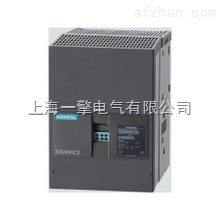设为首页加入收藏联系我们网站首页公司简介公司荣誉产品目录产品中心技术文章新闻动态资料下载供求商机联系我们主营产品： 西门子SIEMENS专业维修，西门子变频器维修，西门子数控维修，西门子触摸屏维修，西门子PLC维修，西门子直流维修电话:021-37683708 传真:021-51687839您现在的位置：网站首页 > 公司产品 >6RA8028-6DV62-0AA06RA8028-6DV62-0AA0 6RA8028-6DV62-0AA0现货《销售态度》：质量保证、诚信服务、及时到位！《销售宗旨》：为客户创造价值是我们永远追求的目标！《服务说明》：现货配送至全国各地含税（17%）含运费！《产品质量》：，*！《产品优势》：专业销售 薄利多销 信誉好，口碑好，价格低，货期短，大量现货,服务周到！为了运行变频器还需要在6RA70中设置以下参数：
参数 USS1(PMU:X300) USS2(CUD1:X172) USS3（CUD2:X162）  P780=2 P790=2 P800=2  P787=0 P797=0 P807=0  P786=3 P796=3 P806=3  P783=6 P793=6 P803=6  P781=2 P791=2 P801=2  P782=127 P792=127 P802=127  P927=6 P927=42 P927=82  P785.1=1 P795.1=1 P805.1=1  P785.2=0 P795.2=0 P805.2=0  P644=2002 P644=6002 P644=9002  P661=2100 P661=6100 P661=9100    本实验采用PMU上的USS接口，因此采用*组参数设定。   P927 = 6  哪种接口修改参数（6=2+4）：PMU + G-SST1；   P780 = 2 设置 G-SST1接口 为USS协议；   P781 = 2 设置 G-SST1过程数据（PZD）的数量 为2；   P782 = 127 设置 G-SST1参数任务（PKW）的数目 由电报长度决定；   P783 = 6 设置 G-SST1接口波特率 为9600；   P785.1 = 0 设置 总线终端负载 OFF （此时，连接线上的终端电阻要为ON）； P785.2 = 0 设置 *个接收字的位10 不具有“由PLC控制”的功能   P661 = 2100 将 接收到*个字的*位B2100  连接到控制字1的Bit 3； P644 = 2002 将 接收到第二个字K2002   连接到主给定P644；

### 留言框

• #### 验证码：

请输入计算结果（填写阿拉伯数字），如：三加四=7
 首页| 关于我们 | 联系方式 | 在线留言| 人才招聘